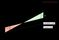# Essays on Break Even

Feeling stuck when writing an essay on Break Even? If you are unable to get started on your task and need some inspiration, then you are in the right place. Break Even essays require a range of skills including understanding, interpretation and analysis, planning, research and writing. To write an effective essay on Break Even, you need to examine the question, understand its focus and needs, obtain information and evidence through research, then build a clear and organized answer. Browse our samples and select the most compelling topic as an example for your own!

We've found 5 essays on Break Even

## information

Contribution Margin and Break Even Analysis

Many factors come into play in determining business success. One of them is the financial factor. For a company to set financial goals it is crucial that its management know in detail the products or services they sale or provide. This is the analysis of …

Break Even
162 views
Words 1467
Pages 6
Break even revised

The break-even point is a business terminology which refers to the level of output or activity wherein a firm’s total revenue exactly equals its total cost. At this point, the business entity covers all its expenses but earns no profit. The operating income is therefore …

Break Even
96 views
Words 106
Pages 1
Breakeven Point: Understanding the Balance between Variable and Fixed Costs in a Company

Breakeven is the point at which the company is not generating either profits or losses. This is the point at which the company is generating just the level of revenue which compensates for both the variable costs and the fixed costs. Variable costs fluctuate with …

Break EvenMicroeconomics
17 views
Words 94
Pages 1
Haven’t found the relevant content? Hire a subject expert to help you with
Essays on Break Even
\$35.80 for a 2-page paper
Break even analysis essay

CASE STUDY Break even analysis which is cost –volume-profit analysis is used to plan and assist in decision making by clarifying the effect of changes in volume and business profitability. In calculating breakeven fixed cost and contribution by unity is calculated; Break-even-point = fixed cost …

Break EvenMicroeconomics
16 views
Words 372
Pages 2
Break Even Analysis

The above breakeven analysis of each variable displays the combination of the variables that result in a break-even position for the Beta project at ABC Corporation. For example, the 6% sales growth rate would result in a break-even for the project if the operating expenses …

Break Even
13 views
Words 75
Pages 1## Find extra essay topics on Essays on Break Even by our writers.

Break-even (or break even), often abbreviated as B/E in finance, (sometimes called point of equilibrium) is the point of balance making neither a profit nor a loss. Any number below the break-even point constitutes a loss while any number above it shows a profit.

### FAQ

What is breakeven point essay?
The break-even point is the point at which a company's revenues equal its expenses, and it is often used as a measure of a company's financial health. The break-even point can be calculated in a number of ways, but the most common method is to divide a company's total fixed costs by its per-unit selling price. For example, if a company's fixed costs are \$1,000 and its per-unit selling price is \$10, then its break-even point is 100 units.The break-even point is important because it represents the point at which a company starts to generate a profit. If a company is selling less than the break-even point, it is losing money. If a company is selling more than the break-even point, it is making a profit. The break-even point is also important because it can be used to calculate a company's financial leverage.The formula for calculating the break-even point is:Break-even point = Total fixed costs / Per-unit selling priceFor example, if a company's fixed costs are \$1,000 and its per-unit selling price is \$10, then its break-even point is 100 units.To calculate a company's financial leverage, you need to know two things: the company's break-even point and its level of debt. The formula for calculating financial leverage is:Financial leverage = Break-even point / Level of debtFor example, if a company's break-even point is 100 units and its level of debt is 50 units, then its financial leverage is 2. This means that for every \$1 of debt, the company has \$2 of equity.The break-even point is a important concept for businesses because it represents the point at which a company starts to generate a profit. The break-even point can be used to calculate a company's financial leverage, which is a measure of a company's ability to generate profits.
How do you explain breakeven?
There are two ways to think about the breakeven point: either in terms of revenue or in terms of units sold. Revenue-wise, the breakeven point is simply the point at which your total revenue (sales) equals your total costs. So if your costs are \$100 and your sales are \$100, you have reached the breakeven point. In terms of units sold, the breakeven point is the number of units you need to sell in order to cover your costs. So if it costs you \$100 to produce one widget and you sell each widget for \$200, you need to sell two widgets to breakeven.
How do you write break-even?
There is no one definitive way to write break-even, as it will vary depending on the specific circumstances and what information is being sought. However, some tips on how to write break-even analysis would include clearly defining what costs and revenue are being considered, using relevant and up-to-date data, and creating clear and easy-to-understand charts and graphs to visualize the information.
Why break-even is so important?